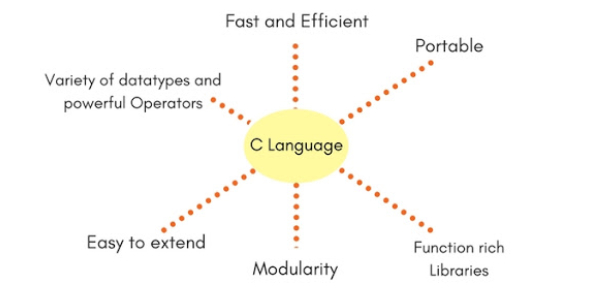# C Language MCQ Exam Trivia! Quiz

15 Questions | Total Attempts: 29Settings.

• 1.
Which of the following is not a valid variable name declaration?
• A.

Int a3;

• B.

Int 3_a;

• C.

Int _3a

• D.

Int a_3;

• 2.
All keywords in C are in?
• A.

Camel Case letters

• B.

Upper Case letters

• C.

Lower Case letters

• D.

None

• 3.
What is the output of this C code?      int main()     {         int i = -5;         int k = i %4;         printf("%d\n", k);     }
• A.

Compile time error

• B.

-1

• C.

1

• D.

None

• 4.
What is the value of x in this C code? void main()     {         int x = 4 *5 / 2 + 9;     }
• A.

6.75

• B.

1.85

• C.

19

• D.

3

• 5.
What is the output of this C code? int main() { int *ptr, a = 10; ptr = &a; *ptr += 1; printf("%d,%d/n", *ptr, a); }
• A.

10,10

• B.

11,10

• C.

10,11

• D.

11,11

• 6.
What is the size of an int data type?
• A.

8 Bytes

• B.

4 Bytes

• C.

Depends on the system/compiler

• D.

Cannot be determined.

• 7.
What is short int in C programming?
• A.

Basic data type of C

• B.

Qualifier

• C.

Short is the qualifier and int is the basic datatype

• D.

All of the mentioned.

• 8.
Which of the datatypes have a size that is variable?
• A.

Double

• B.

Int

• C.

Struct

• D.

Float

• 9.
What is the output of this C code? int main() { int x = 1; int y = x == 1 ? getchar(): 2; printf("%d\n", y); }
• A.

Compile time error

• B.

Whatever character getchar function returns

• C.

Ascii value of character getchar function returns

• D.

2

• 10.
#include is called
• A.

Preprocessor directive

• B.

File inclusion directive

• C.

Inclusion directive

• D.

None of the mentioned

• 11.
C preprocessors can have compiler-specific features.
• A.

True

• B.

False

• C.

Depends on the standard

• D.

Depends on the platform

• 12.
Which of the following are C preprocessors?
• A.

#ifdef

• B.

#define

• C.

#endif

• D.

All of the mentioned.

• 13.
#include statement must be written:
• A.

Before main()

• B.

After main()

• C.

Before any scanf/printf

• D.

It can be written anywhere

• 14.
The C-preprocessors are specified with _________symbol.
• A.

#

• B.

\$

• C.

" "

• D.

None of the mentioned

• 15.
Does this compile without error? int main() { for (int k = 0; k < 10; k++); return 0; }
• A.

Yes

• B.

No

• C.

Depends on the C standard implemented by compilers

• D.

None of the mentioned

Related TopicsBack to top